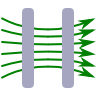Currents and their Magnetic Fields

Ampère's law, $$\nabla\times\mathbf{B}\left(\mathbf{r}\right)=\mu_{0}\mathbf{j}\left(\mathbf{r}\right)$$ relates the magnetic induction $$\mathbf{B}\left(\mathbf{r}\right)$$ to the current distribution $$\mathbf{j}\left(\mathbf{r}\right)$$. In this section we will learn the basic techniques to calculate the magnetic induction for a given current distribution. We will use integral methods like the law of Biot-Savart or differential methods for certain symmetries to solve Ampère's law directly. If you have some nice problems that would fit here or you found some errors, please do not hesitate to contact us!

The Magnetic Field of a Hollow WireWhat happens if we take a wire, drill a hole in it and set some current in motion? Will we encounter a magnetic field?

The Magnetic Field due to a Thin Infinite WireWe will directly use the Biot-Savart law to calculate the magnetic field of a thin wire via integration. The problem shall provide us with some intuition of Biot-Savart which we will also derive.

Providing a Homogeneous Magnetic Field - The Helmholtz CoilHelmholtz coils are a devices that can provide a very homogeneous magnetic field. Although the principle is very old, it is still applied in state-of-the-art experiments. In this problem you will learn how the special characteristics of such coils are achieved.

The Magnetic Field of an Infinite WireOne of the basic problems of magnetostatics is the infinite wire. Using the several symmetries of the current distribution we will be able to not only find the magnetic field - we will also verify the "right-hand rule".

Spin is not a Classical RotationIn an attempt to explain the electron spin in a classical model, one can assume that it is just a rotating charged particle causing a magnetic moment. However, such an approach results in contradictions which renders a classical explanation of the intrinsic magnetic moment of an electron obsolete.

A Cylinder Shell Current and its Magnetic FieldAmpère's law is hard to solve in general. What if certain symmetries of a current distribution are present? We may find much simpler differential equations which might be integrated directly. Find out what to do in rotational and translational symmetry!